# 二维码 QRCode 标准阅读¶

## 基础描述及结构（6.1、6.3）¶

### 基础描述（5.3、6.1）¶

• 块位置：左上角为原点 (0, 0) 向下x+，向右y+
• 版本表示：Version V-E（其中V是版本号，E是纠错等级）
• 数据表示：黑块-1 白块-0（可以带背景全部反色）
• 大小：从版本1到版本40依次是 21x21 ～ 177x177（每增加一个版本，边长增加4）
• 支持的最多字符数（版本40）
• 数字模式：7089
• 字母模式：4296
• 字节模式：2953
• 日文模式：1817
• 纠错等级允许的恢复比例
• L：7%
• M：15%
• Q：25%
• H：30%

### 二维码结构（6.3）¶

• 功能图案（function patterns）
• 特征符（finder pattern）7x7黑圈 5x5白圈 3x3黑块
• 分割线（separator）在特征符周围的一圈全白区域
• 时序图案（timing patterns）第7行第7列的两条黑白条纹
• 对齐图案（alignment patterns）版本1无，版本2-6 1个，版本7-13 6个……（附录E）
• 静默区（quiet zone）至少4个单位宽
• 编码区域（encoding region）
• 格式信息（format information）左上角分割线外一圈，左下角分割线右侧，右上角分割线下侧
• 版本信息（version information）版本7后才有，在左下分割线上侧，右上分割线左侧
• 数据及纠错码区域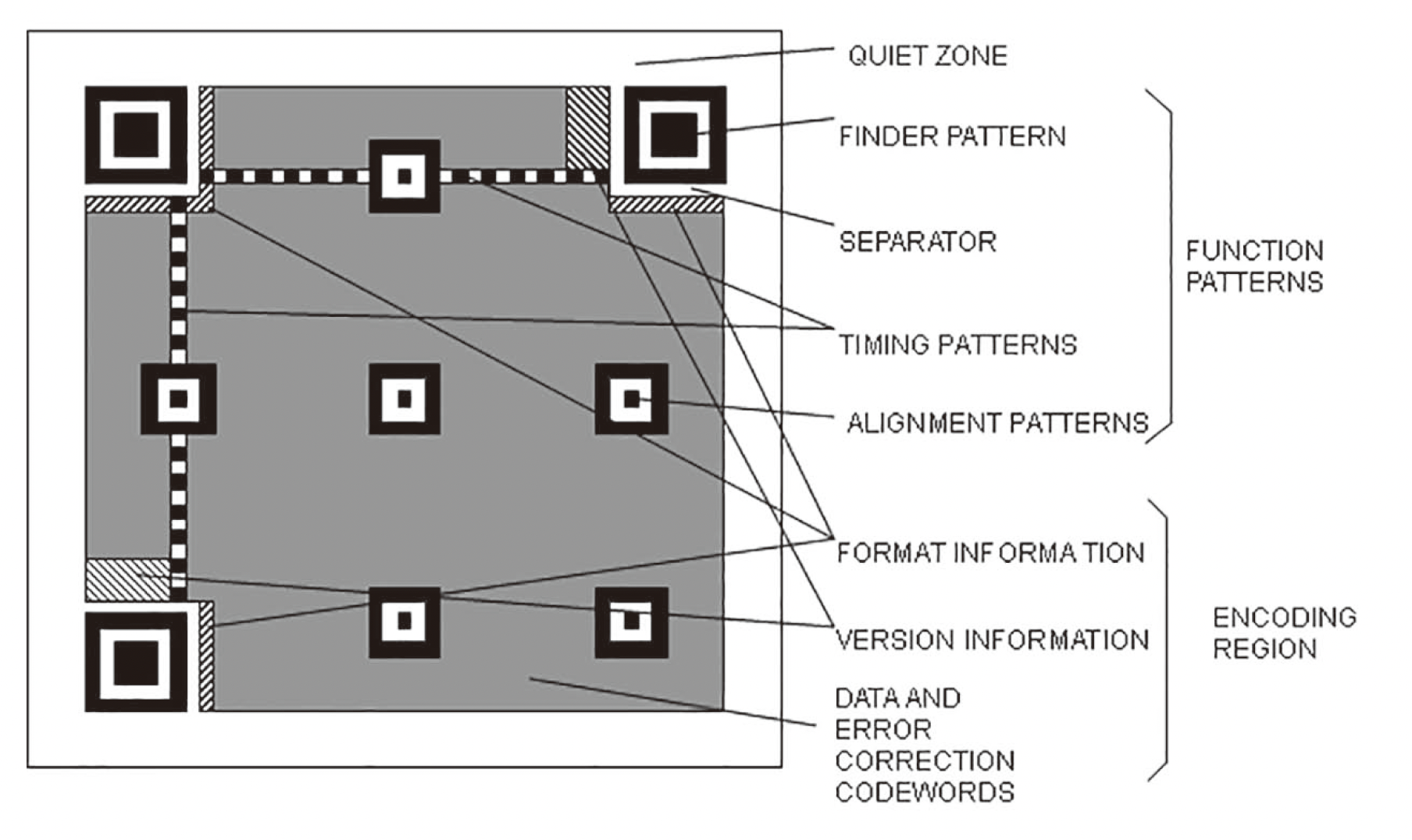## 数据编码（7.4）¶

### 数据序列（7.4.1）¶

• （4 bits）ECI 模式标识符
• （8/16/24 bits）ECI Designator

• （4 bits）模式标识符
• 字符数量标识符（长度见下第二个表）
• 数据比特流

ECI 0111

1～9 10 9 8 8
10～26 12 11 16 10
27～40 14 13 16 12

### ECI 模式（7.4.2）¶

ECI 模式即使用某些特定的字符映射来把字符转换为比特流

ECI Assignment Value Codewords values
000000 ～ 000127 0xxxxxxx
000000 ～ 016383 10xxxxxx xxxxxxxx
000000 ～ 999999 110xxxxx xxxxxxxx xxxxxxxx

• ECI 标识符：0111
• ECI 编号：00001001
• 字节模式标识符：0100
• 字符数量：00000101（5个字符）
• 数据：10100001 10100010 10100011 10100100 10100101

• 需要编码的数据：ABC\123456
• 数据流中十六进制（字节模式标识符0100后）：41 42 43 5C 5C 31 32 33 34 35 36
• 需要编码的数据：ABC<后接 ECI 123456 下的数据……>
• 数据流中十六进制（字节模式标识符0100后）：41 42 43 5C 31 32 33 34 35 36 ……

### 数字模式（7.4.3）¶

• 数据内容： 01234567（保留开头0）
• 数据流部分：
• 数字模式标识符： 0001
• 数量标识符： 0000001000（8，且版本1下规定为 10 bits）
• 数据：
• 012 -> 0000001100
• 345 -> 0101011001
• 67 -> 1000011
• 完整数据比特流： 0001 0000001000 0000001100 0101011001 1000011

$B=M+C+10\times\lfloor\frac{D}{3}\rfloor+R$

### 字母数字模式（7.4.4）¶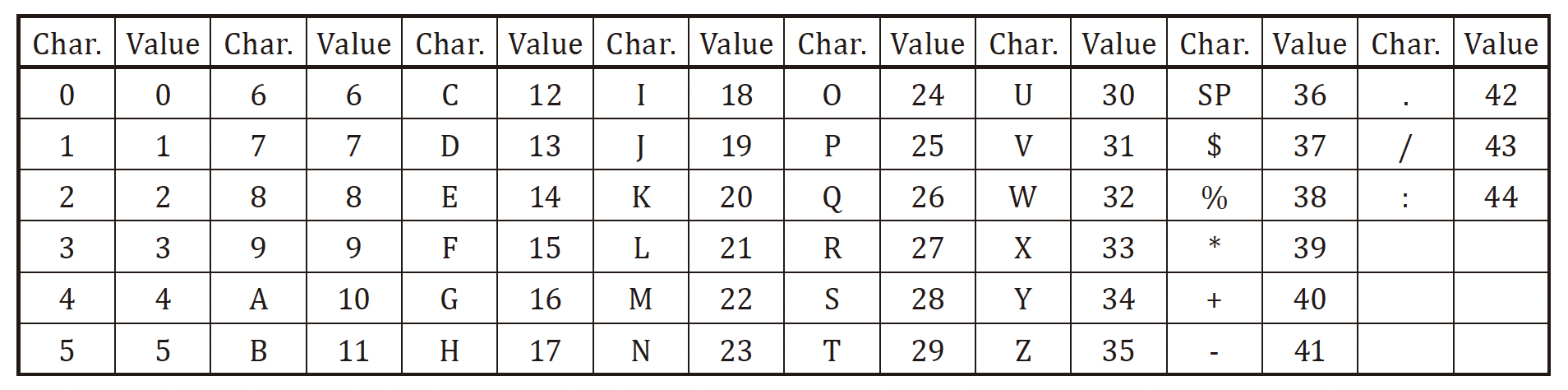• 字母数字模式标识符： 0010
• 数量标识符： 000000101（5，且版本1下规定长度为9）
• 数据：AC-42 -> 10 12 41 4 2 -> (10 12)(41 4)(2)
• 10 12 -> 10*45+12=462 -> 00111001110
• 41 4 -> 41*45+4=1849 -> 11100111001
• 2 -> 2 -> 000010

$B=M+C+11\times\lfloor\frac{D}{2}\rfloor+6\times(D\bmod 2)$

### 字节模式（7.4.5）¶

Latin-1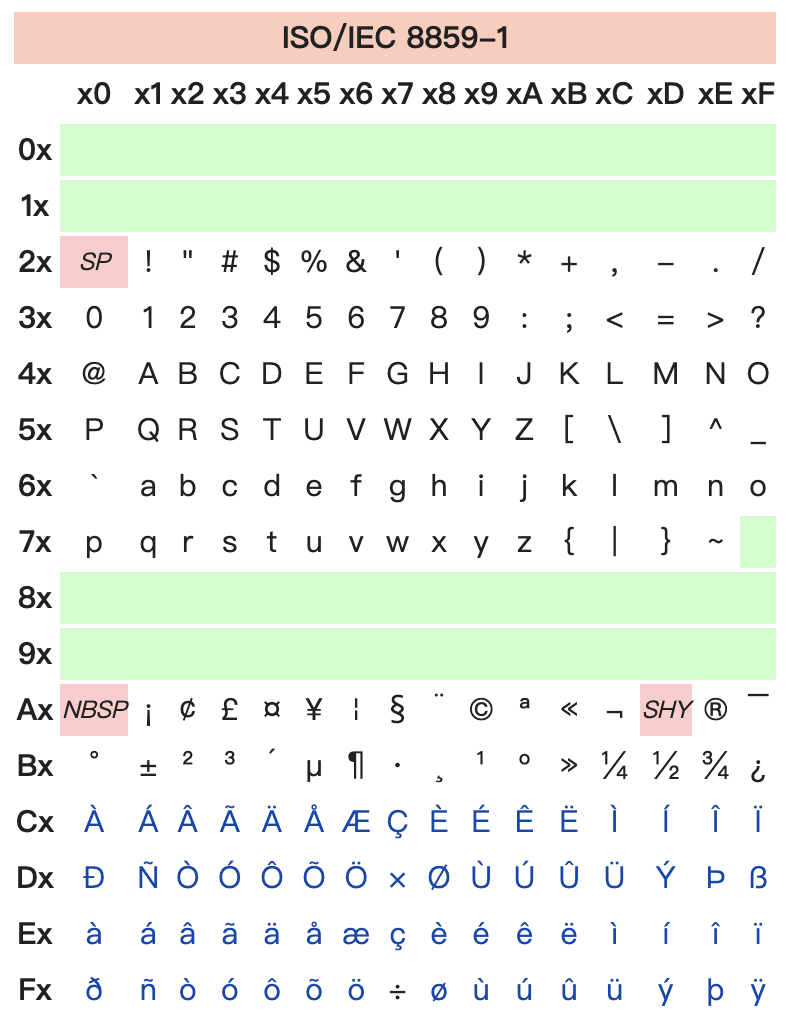$B=M+C+8\times D$

### 混合模式（7.4.7）¶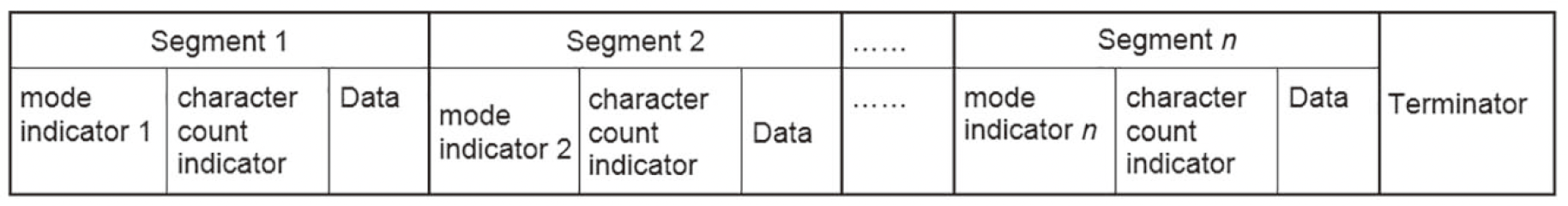• 数字模式：
• 标识符： 0001
• 数量标识符： 0000000011（3，长度10）
• 数据：123 -> 0001111011
• 字节模式：测试 -> E6 B5 8B / E8 AF 95
• 标识符： 0100
• 数量标识符： 00000110（6，长度8）
• 数据：
• 测 -> 11100110 10110101 10001011
• 试 -> 11101000 10101111 10010101

### 结束符（7.4.9）¶

1. 首先先用 0 补充比特流长度到 8 的整数倍
2. 然后用 1110110000010001 交替填补到二维码数据容量

## 纠错码编码（7.5）¶

### 纠错容量（7.5.1）¶

$e+2t\leq d-p$

• $$p=3$$：版本 1-L
• $$p=2$$：版本 1-M、2-L
• $$p=1$$：版本 1-Q、1-H、3-L
• $$p=0$$：其他所有版本

### 生成纠错码（7.5.2）¶

#### 生成多项式（Annex A）¶

$g(x)=(x-\alpha^0)(x-\alpha^1)\cdots(x-\alpha^{n-1})$

$$\alpha^0 = 1;\ \alpha^1=2;\ \alpha^2=4;\ \cdots;\ \alpha^7=128$$

$$\alpha^8=256\bmod 285=256\oplus 285=29;\ \alpha^9=29\times2=58;\ \cdots$$

def alpha(k):
res = 1
for _ in range(k):
res *= 2
if res >= 256:
res ^= 285
return res


#### 生成纠错码¶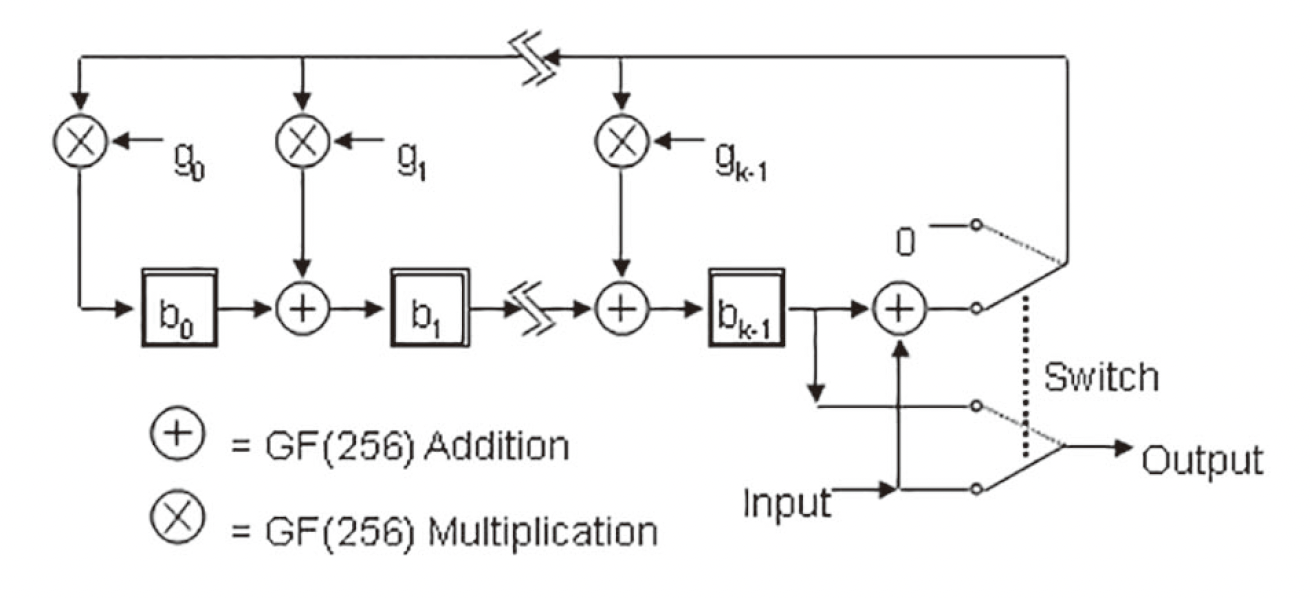$16x^{25}+32x^{24}+123x^{23}+114x^{22}+39x^{21}+236x^{19}+\\\\17x^{18}+236x^{17}+17x^{16}+236x^{15}+17x^{14}+236x^{13}+\\\\17x^{12}+236x^{11}+17x^{10}+236x^{9}+17x^{8}+236x^{7}$

$x^{25}+\alpha^{87}x^{24}+\alpha^{229}x^{23}+\alpha^{146}x^{22}+\alpha^{149}x^{21}+\\\\\alpha^{238}x^{20}+\alpha^{102}x^{19}+\alpha^{21}x^{18}$

$\alpha^4x^{25}+\alpha^{91}x^{24}+\alpha^{233}x^{23}+\alpha^{150}x^{22}+\alpha^{153}x^{21}+\\\\\alpha^{242}x^{20}+\alpha^{106}x^{19}+\alpha^{25}x^{18}$

$16x^{25}+163x^{24}+243x^{23}+85x^{22}+146x^{21}+\\\\176x^{20}+52x^{19}+3x^{18}$

$131x^{24}+136x^{23}+197x^{22}+181x^{21}+216x^{19}+18x^{18}+\\\\236x^{17}+17x^{16}+236x^{15}+17x^{14}+236x^{13}+\\\\17x^{12}+236x^{11}+17x^{10}+236x^{9}+17x^{8}+236x^{7}$

$188x^6+247x^5+62x^4+248x^3+53x^2+170x+224$

>>> from reedsolo import RSCodec, ReedSolomonError
>>> rsc = RSCodec(7)
>>> list(rsc.encode([16, 32, 123, 114, 39, 0, 236, 17, 236, 17, 236, 17, 236, 17, 236, 17, 236, 17, 236]))
[16, 32, 123, 114, 39, 0, 236, 17, 236, 17, 236, 17, 236, 17, 236, 17, 236, 17, 236, 188, 247, 62, 248, 53, 170, 224]
>>> list(rsc.encode([16, 32, 123, 114, 39, 0, 236, 17, 236, 17, 236, 17, 236, 17, 236, 17, 236, 17, 236]))[-7:]
[188, 247, 62, 248, 53, 170, 224]


## 剩余步骤（7.6~7.10）¶

### 合成序列（7.6）¶### 填充数据（7.7）¶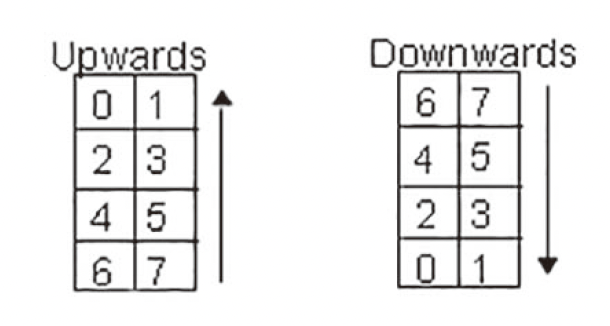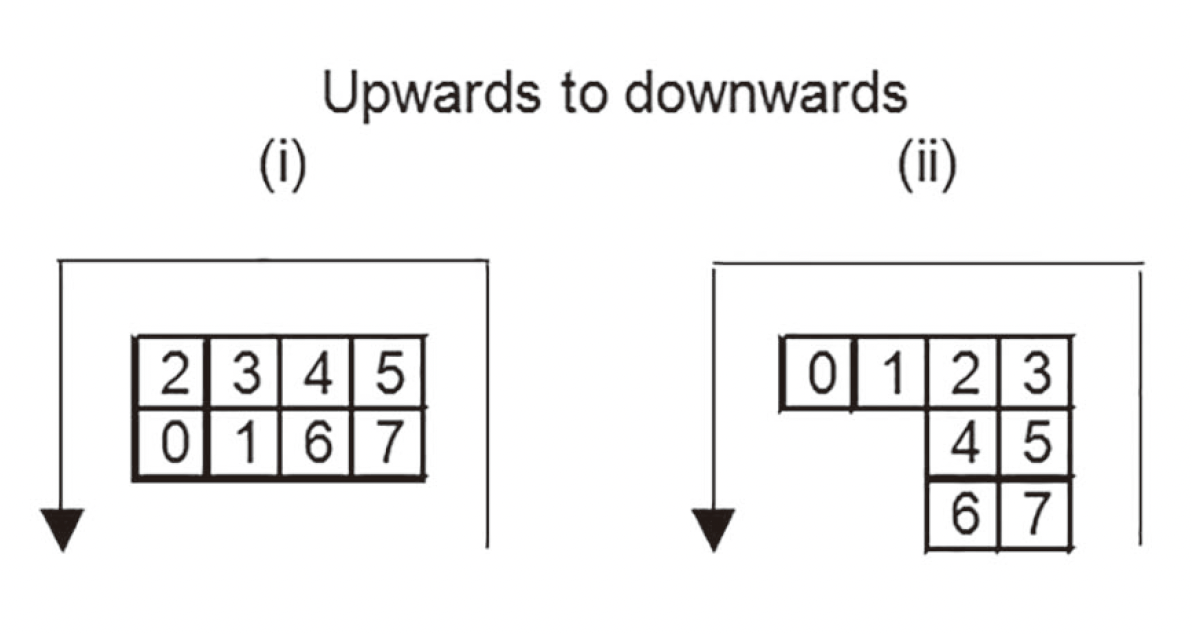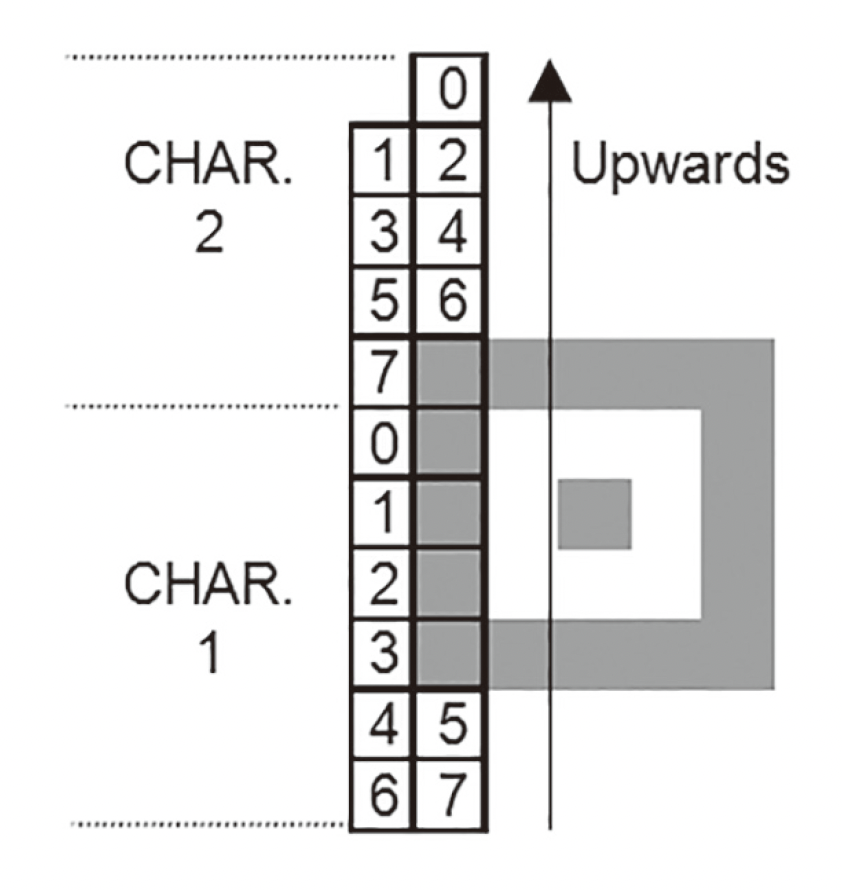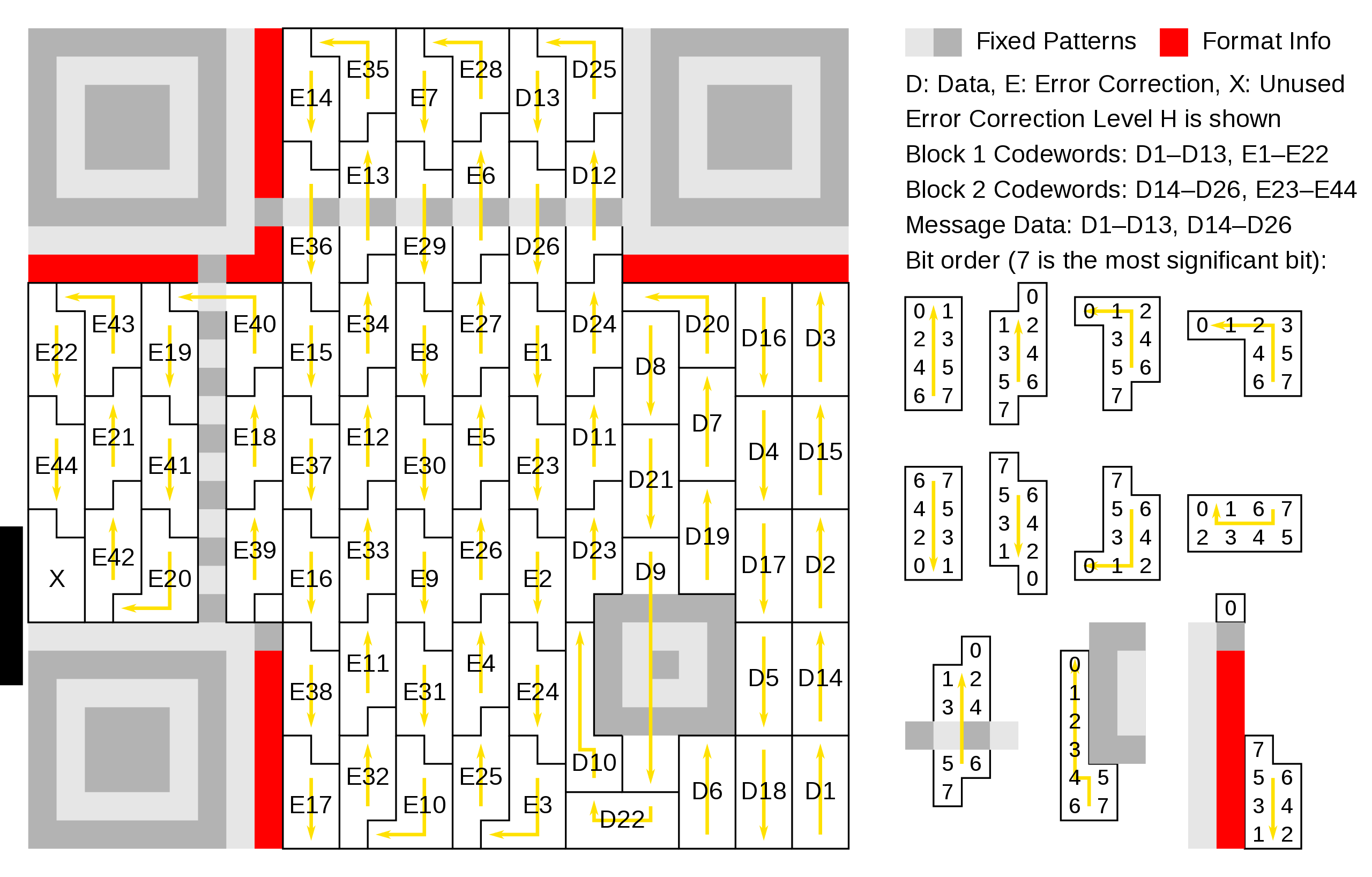### 掩码遮盖（7.8）¶

QR 码一共有 8 种掩码，每个掩码有一个 3 bits 的编号，和一个生成公式。这个公式用来生成掩码图样，以左上为原点，向右、下为正方向，坐标满足这个公式的点在图样中是黑色（1），不满足的是白色（0）。在版本 1 中的掩码图像表现为：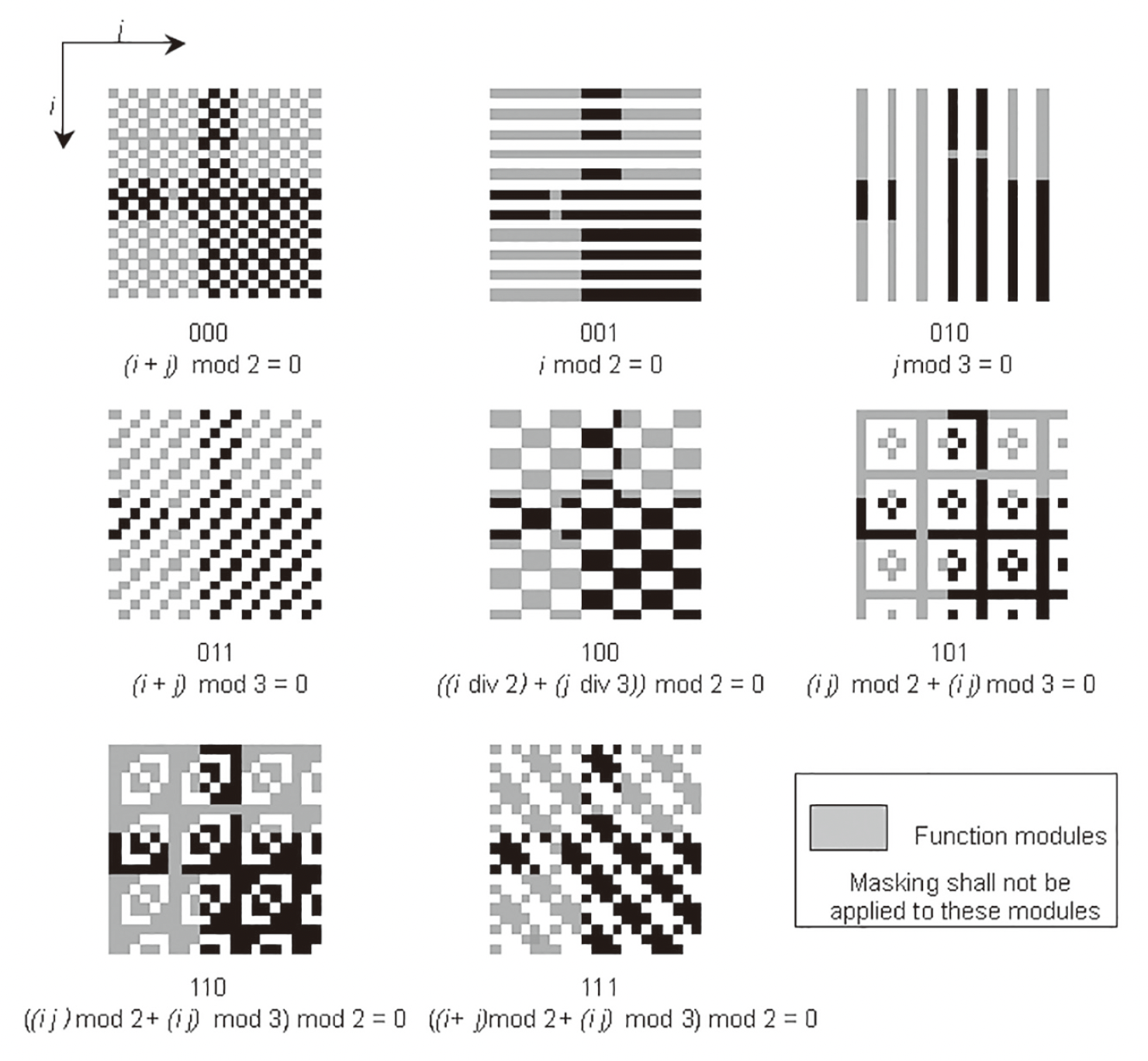#### 计算损失分数（7.8.3）¶

• 相邻一行或一列内出现连续五个相同颜色块时损失分 +3，之后连续块数每加一，损失分 +1
• 寻找内部颜色相同的 2*2 的块，每出现一个损失分 +3
• 在每行和每列中寻找 1011101000000001011101 ，每出现一个损失分 +40
• 评估黑色块占全部块数的比例，如果在 45%~55% 间则不增加损失分，在 40%~45%、55%~60% 间则损失分 +10，在 35%~40%、60%~65% 间则损失分 +10*2，以此类推

### 格式信息（7.9）¶

QRCode 的格式信息是 15 bits 的序列，其中前 5 位是数据，后 10 位是针对格式信息的纠错码（由 (15, 5) BCH 码生成）

5 bits 的数据前 2 位是纠错等级标识符，分别是 L -> 01 、M -> 00 、Q -> 11 、H -> 10

#### 生成纠错码（Annex C）¶

• 纠错等级 M，掩码编号 101
• 5 bits 数据： 00101
• 写为多项式： $$x^2+1$$
• 整体乘 $$x^{10}$$$$x^{12}+x^{10}$$
• 除以 $$G(x)$$： 商 $$x^2$$，余数 $$x^7+x^6+x^4+x^3+x^2$$
• 余数转为 10 bits 纠错码： 0011011100
• 加上原数据： 001010011011100
• 异或 101010000010010100000011001110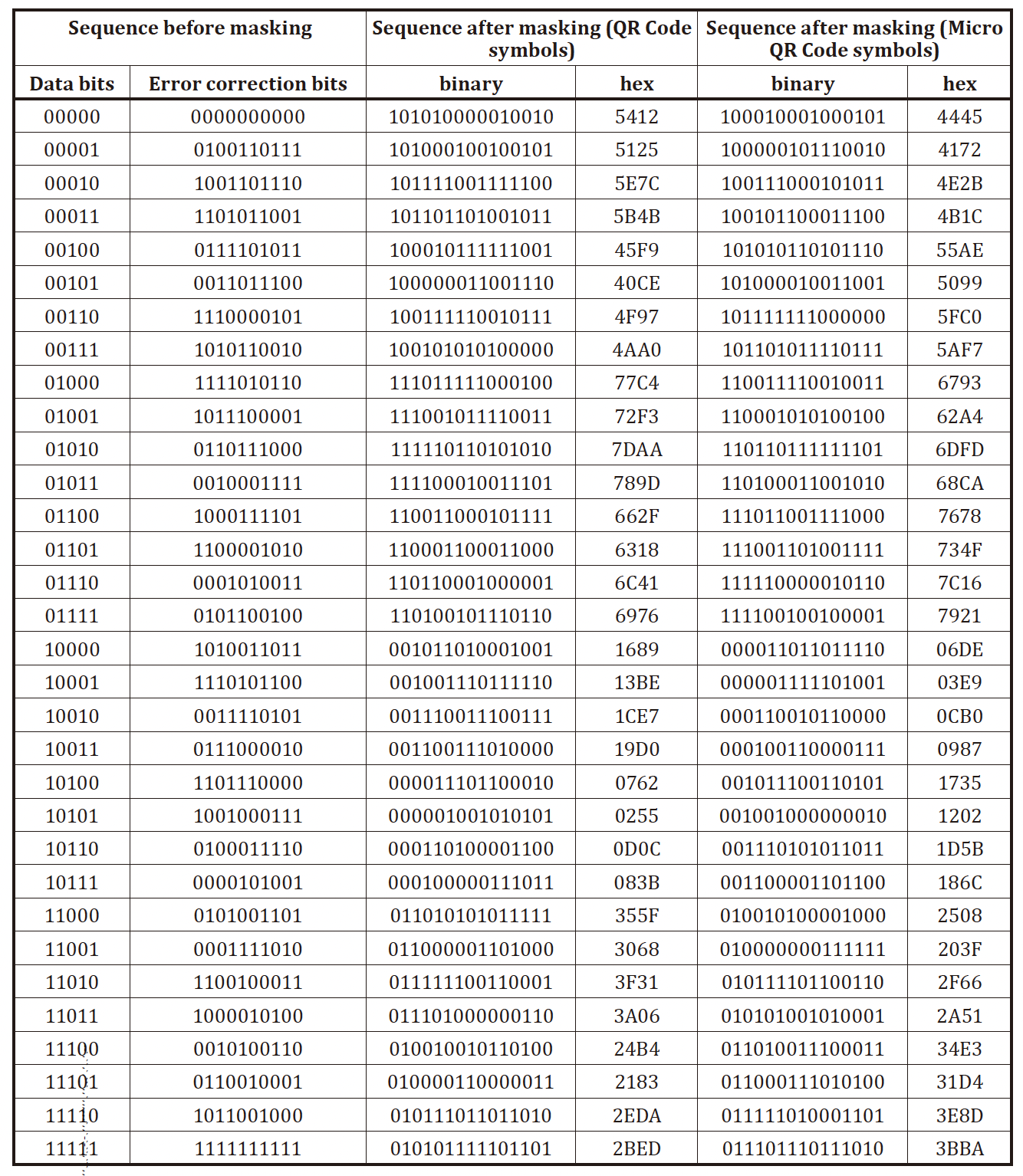#### 填入二维码¶### 版本信息（7.10）¶

#### 生成纠错码（Annex D）¶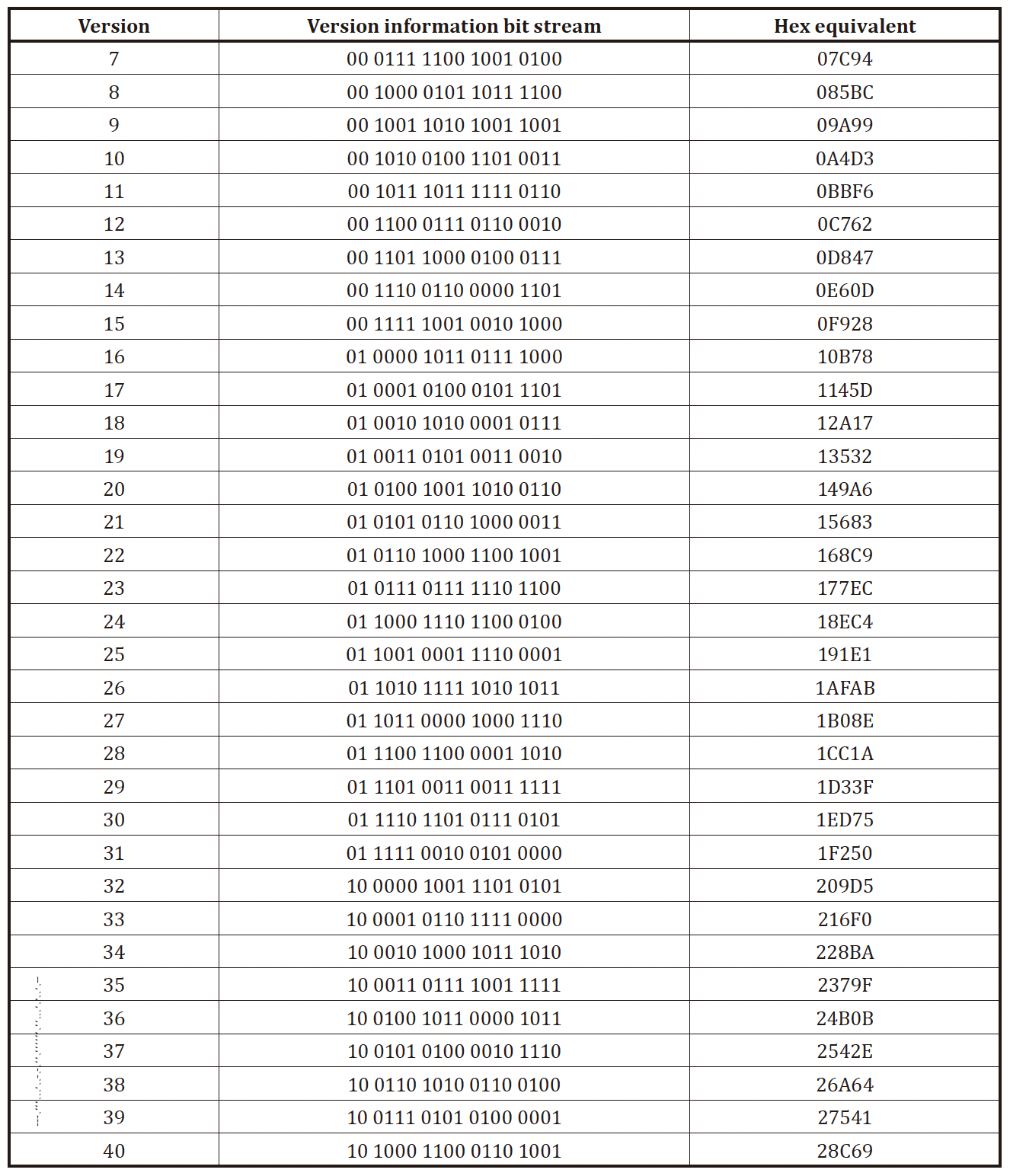#### 填入二维码¶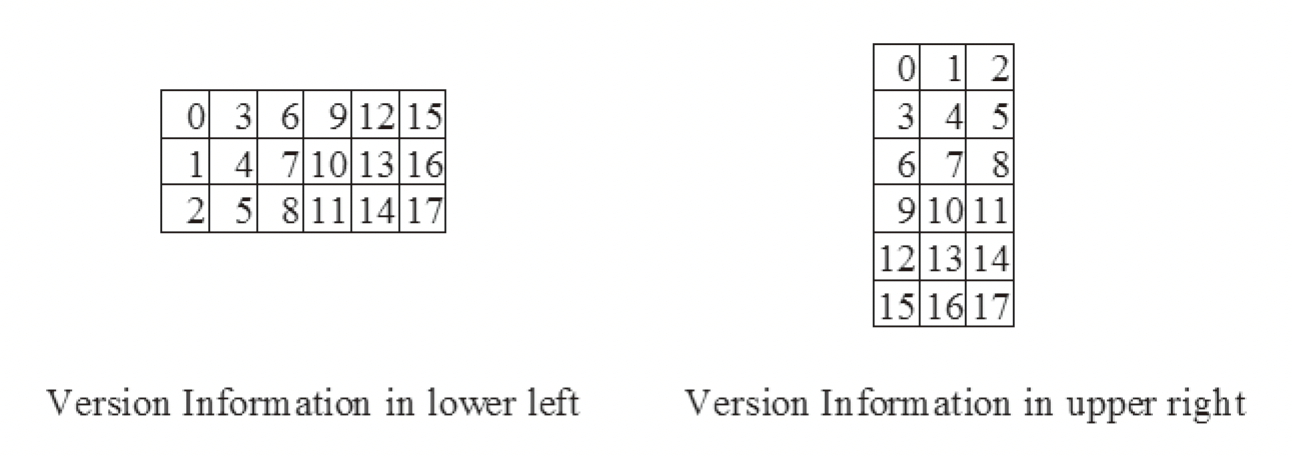## 解码（11、Annex B）¶

1. 定位并获取图像中的二维码，并把图像中的黑白块提取为 1 和 0
2. 读取格式信息
• 释放掩码 101010000010010
• 进行纠错
• 如果纠错失败则将二维码镜面对称再尝试
3. 读取版本信息（如果有的话）
4. 读取格式信息中的掩码编号，并释放掩码
5. 读取并恢复数据字和纠错字
6. 纠错，如果检测到了错误就纠正
7. 把数据字解码得到结果

### 纠错（Annex B）¶

Annex B 讲的纠错过程很简略，而且符号说明不全，很难看懂

$s(x)=\sum_{i=0}^{n-1}c_ix^i=c_{n-1}x^{n-1}+c_{n-2}x^{n-2}+\cdots+c_0$

$g(x)=\prod_{i=0}^{n-k-1}(x-\alpha^i)$

$r(x)=s(x)+e(x),\quad e(x)=\sum_{i=0}^{n-1}e_ix^i$

$e(x)=\sum_{k=0}^{\nu-1}e_{i_k}x^{i_k}$

#### 计算典型值¶

$S_j=r(\alpha^j)=s(\alpha^j)+e(\alpha^j)=e(\alpha^j)=\sum_{k=0}^{\nu-1}e_{i_k}(\alpha^j)^{i_k},j=0,1,\dots,n-k-1$

$S_j=\sum_{k=0}^{\nu-1}Y_kX_k^j$

$\begin{bmatrix} X_0^0 &X_2^0 &\cdots &X_{\nu-1}^0\\\\ X_0^1 &X_1^1 &\cdots &X_{\nu-1}^1\\\\ \vdots &\vdots & &\vdots\\\\ X_0^{n-k-1} &X_1^{n-k-1} &\cdots &X_{\nu-1}^{n-k-1} \end{bmatrix} \begin{bmatrix} Y_0\\\\Y_1\\\\\vdots\\\\Y_{\nu-1} \end{bmatrix}= \begin{bmatrix} S_0\\\\S_1\\\\\vdots\\\\S_{n-k-1} \end{bmatrix}$

#### 错误定位多项式¶

$\Lambda(x)=\prod_{k=0}^{\nu-1}(1-xX_k)=1+\Lambda_1x+\Lambda_2x^2+\cdots+\Lambda_\nu x^\nu$

$Y_kX_k^{j+\nu}\Lambda(X_k^{-1}) =0$
$Y_{k}X_{k}^{j+\nu }(1+\Lambda _{1}X_{k}^{-1}+\Lambda _{2}X_{k}^{-2}+\cdots +\Lambda _{\nu }X_{k}^{-\nu })=0$
$Y_{k}X_{k}^{j+\nu }+\Lambda _{1}Y_{k}X_{k}^{j+\nu }X_{k}^{-1}+\Lambda _{2}Y_{k}X_{k}^{j+\nu }X_{k}^{-2}+\cdots +\Lambda _{\nu }Y_{k}X_{k}^{j+\nu }X_{k}^{-\nu }=0$
$Y_{k}X_{k}^{j+\nu }+\Lambda _{1}Y_{k}X_{k}^{j+\nu -1}+\Lambda _{2}Y_{k}X_{k}^{j+\nu -2}+\cdots +\Lambda _{\nu }Y_{k}X_{k}^{j}=0$

$\sum_{k=0}^{\nu-1}\left(Y_{k}X_{k}^{j+\nu }+\Lambda _{1}Y_{k}X_{k}^{j+\nu -1}+\Lambda _{2}Y_{k}X_{k}^{j+\nu -2}+\cdots +\Lambda _{\nu }Y_{k}X_{k}^{j}\right)=0$

$\left(\sum _{k=1}^{\nu }Y_{k}X_{k}^{j+\nu }\right)+\Lambda _{1}\left(\sum _{k=1}^{\nu }Y_{k}X_{k}^{j+\nu -1}\right)+\cdots +\Lambda _{\nu }\left(\sum _{k=1}^{\nu }Y_{k}X_{k}^{j}\right)=0$

$S_{j+\nu}+\Lambda_1S_{j+\nu-1}+\cdots+\Lambda_\nu S_k=0$

$$S_{j+\nu}$$ 移到右边，并展开所有 $$j$$ 可以得到矩阵形式：

$\begin{bmatrix} S_{0}&S_{1}&\cdots &S_{\nu-1}\\\\S_{1}&S_{2}&\cdots &S_{\nu}\\\\\vdots &\vdots &&\vdots \\\\S_{\nu-1}&S_{\nu}&\cdots &S_{2\nu -2} \end{bmatrix} \begin{bmatrix}\Lambda _{\nu }\\\\\Lambda _{\nu -1}\\\\\vdots \\\\\Lambda _{1} \end{bmatrix} = \begin{bmatrix} -S_{\nu}\\\\-S_{\nu +1}\\\\\vdots \\\\-S_{2\nu-1 } \end{bmatrix}$